# Samacheer Kalvi 9th Science Solutions Chapter 6 Light

## Tamilnadu Samacheer Kalvi 9th Science Solutions Chapter 6 Light

### Samacheer Kalvi 9th Science Light Textbook Exercises

Chapter 6 Light Question 1.
A ray of light passes from one medium to another medium. Refraction takes place when angle of incidence is …………..
(a) 0°
(b) 45°
(c) 90°
(b) 45°

Light 9th Class Question 2.
…………. is used as reflectors in torchlight.
(a) Concave mirror
(b) Plane mirror
(c) Convex mirror
(a) Concave mirror

9th Science Light Question 3.
We can create enlarged, virtual images with ………………
(a) Concave mirror
(b) Plane mirror
(c) Convex mirror
(a) Concave mirror

9th Science Reflection Of Light Exercise Question 4.
When the reflecting surface is curved outwards the mirror formed will be
(a) concave mirror
(b) convex mirror
(c) plane mirror
(b) convex mirror

9th Science Reflection Of Light Question 5.
When a beam of white light passes through a prism it gets
(a) reflected
(b) only deviated
(c) deviated and dispersed
(a) Reflected

Unit 6 Light Question 6.
The speed of light is maximum in
(a) vacuum
(b) glass
(c) diamond
(a) vacuum

II. True or False – If false give the correct answer.

1. The angle of deviation depends on the refractive index of the glass – True.
2. If a ray of light passes obliquely from one medium to another, it does not suffer any deviation – False.
Correct Statement: When light travels from one medium to another, it suffers deviation.
3. The convex mirror always produces a virtual, diminished and erect image of the object – True.
4. When an object is at the centre of curvature of concave mirror the image formed will be virtual and erect – False.
Correct Statement: The image formed is real, inverted and same size of the object.
5. The reason for brilliance of diamonds is total internal reflection of light – True.

III. Fill in the blanks.

1.  In going from a rarer to denser medium, the ray of light bends …………….
2. The mirror used in search light is ………………
3. The angle of deviation of light ray in a prism depends on the angle of ……………..
4. The radius of curvature of a concave mirror whose focal length is 5 cm is …………….
5. Large ………… mirrors are used to concentrate sunlight to produce heat in solar furnaces.

1. towards normal
2. concave mirror
3. prism and angle of incident
4. 10 cm
5. concave

IV. Match the following.

 1. Ratio of height of image to height of object (a) Concave Mirror 2. Used in hairpin bends in mountains (b) Total Internal Reflection 3. Coin inside water appearing slightly raised (c) Magnification 4. Mirage (d) convex Mirror 5. Used as Dentist’s mirror (e) Refraction

1. (c)
2. (d)
3. (e)
4. (b)
5. (a)

V. Assertion & Reason.

Mark the correct choice as:
(a) If both assertion and reason are true and reason is the correct explanation.
(b) If both assertion and reason are true and reason is not the correct explanation.
(c) If assertion is true but reason is false.
(d) If assertion is false but reason is true.

Light Chapter Of Class 6 Pdf Question 1.
Assertion: For observing the traffic at a hairpin bend in mountain paths a plane mirror is
preferred over convex mirror and concave mirror.
Reason : A convex mirror has a much larger field of view than a plane mirror or a concave mirror.
(d) If assertion is false but reason is true.

Samacheer Kalvi 9th Science Question 2.
Assertion : Incident ray is directed towards the centre of curvature of spherical mirror.
After reflection it retraces its path.
Reason : Angle of incidence i = Angle of reflection r = 0°.
(b) If both assertion and reason are true and reason is not the correct explanation.

Kalvi Guru 9th Science Question 1.
According to cartesian sign convention, which mirror and which lens has negative focal length?
Concave mirror is having a negative focal length.

Samacheer Kalvi Guru 9th Science Question 2.
Name the mirror(s) that can give (i) an erect and enlarged image, (II) same sized, inverted image.
Concave mirror

9th Class Science Reflection Of Light Exercise Question 3.
If an object is placed at the focus of a concave mirror, where is the image formed? Image will be formed at infinity as real and inverted.

9th Science Chapter 11 Reflection Of Light Exercise Question 4.
Why does a ray of light bend when it travels from one medium to another?
The bending of light rays when they pass obliquely from one medium to another medium is called refraction of light.

Light rays get deviated from their original path while entering from one transparent medium to another medium of different optical density. This deviation (change in direction) in the path of light is due to the change in velocity of light in the different medium. The velocity of light depends on the nature of the medium in which it travels.

Questions On Reflection Of Light Class 9 Question 5.
What is speed of light in vacuum?
The speed of light in vacuum is known to be almost exactly 300,000 km per second. In 1665 the Danish astronomer Ole Roemer first estimated the speed of light by observing one of the twelve moons of the planet Jupiter.

Std 9 Science Reflection Of Light Question 6.
Concave mirrors are used by dentists to examine teeth. Why?
As a dentist’s head mirror: You would have seen a circular mirror attached to a band tied to the forehead of the dentist/ENT specialist. A parallel beam of light is made to fall on the concave mirror; this mirror focuses the light beam on a small area of the body (such as teeth, throat etc.).

9th Class Science Chapter 11 Reflection Of Light Exercise Question 1.
(a) Complete the diagram to show how a concave mirror forms the image of the object.
(b) What is the nature of the image?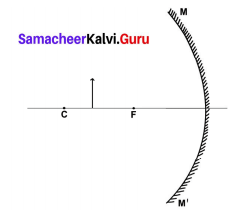Solution:
(a)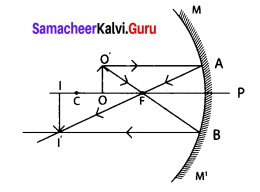(b) magnified, real and inverted.

Reflection Of Light Class 9 Exercise Question 2.
Pick out the concave and convex mirrors from the following and tabulate them
Rear-view mirror, Dentist’s mirror, Torch-light mirror, Mirrors in shopping malls, Make-up mirror.

 Concave mirror Convex mirror Dentist’s mirror Rear view mirror Torch light mirror Mirrors in shopping malls Make-up mirror

Question 3.
State the direction of incident ray which after reflection from a spherical mirror retraces its path. Give reason for your answer.
When incident ray is directed towards the centre of curvature, at all the points of spherical mirror, the ray is always normal. Therefore, angle of incidence i = Angle of reflection r = 0°.

Question 4.
What is meant by magnification? Write its expression. What is its sign for real image and virtual image?
Magnification produced by a spherical mirror gives how many times the image of an object is magnified with respect to the object size.
It can be defined as the ratio of the height of the image (hi) to the height of the object (ho).
Magnification = m = $$\frac{h_{i}}{h_{o}}=\frac{\text { height of the image }}{\text { height of the object }}$$

• for real image it is negative,
• for virtual image it is positive.

Question 5.
Write the spherical mirror formula and explain the meaning of each symbol used in it.
The expression relating the distance of the object u, distance of image v and focal length/of a spherical mirror is called the mirror equation. It is given as:
Mirror formula: $$\frac{1}{f}=\frac{1}{u}+\frac{1}{v}$$
Here, f – focal length of spherical mirror; u – distance of the objective; v – distance of the image.

Question 1.
(a) Draw ray diagrams to show how the image is formed, using a concave mirror when the position of object is

1.  at C
2.  between C and F
3.  between F and P of the mirror.

(1) At the centre of curvature C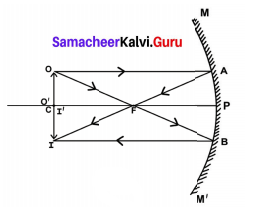(2) Between C and F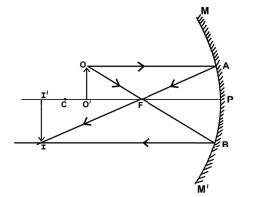(3) Between the focus F and the Pole P of the mirror.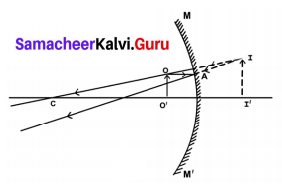(b) Mention the position and nature of image in each case.

 Position of object Position of Image Nature of Image At the centre of curvature C AtC Real and Inverted Between C and F Beyond C Real and inverted Between F and P Behind the mirror Virtual and Erect

Question 2.
Explain with diagrams how refraction of incident light takes place from
(a) rarer to denser medium
(b) denser to rarer medium
(c) normal to the surface separating the two media.
(a) rarer to denser medium:
When a ray of light travels from optically rarer medium to optically denser medium, it bends towards the normal.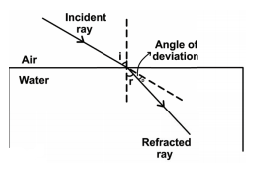(b) denser to rarer medium:
When a ray of light travels from an optically denser medium to an optically rarer medium it bends away from the normal.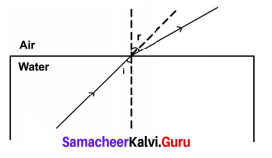(c) normal to the surface separating the two media:
A ray of light incident normally on a denser medium, goes without any deviation.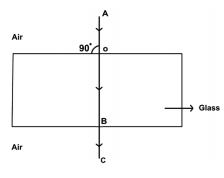IX. Numerical problems.

Question 1.
A concave mirror produces three times magnified real image of an object placed at 7 cm in front of it. Where is the image located? (21 cm in front of the mirror)
Given: hi = 3ho
u = 7 cm
Solution:

1. m = $$\frac{h_{i}}{h_{O}} \Rightarrow \frac{3 h o}{h_{O}}$$ ∴ m = 3.
2. m = – $$\frac{v}{u}$$
v = – m × u
= – 3 × 7 cm = – 21 cm.
Hence, Real, inverted and magnified image will be formed at 21 cm in front of the mirror.

Question 2.
Light enters from air into a glass plate having refractive index 1.5. What is the speed of light in glass? (2 × 108 ms– 1)
Given: Refractive index (µ) = 1.5
Speed of light in vacuum (c) = 2 × 108ms– 1
Speed of light in glass (υ) = ?
Solution: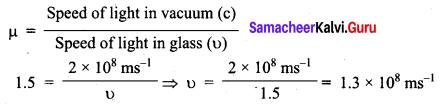∴ Speed of light in glass = 1.3 × 108 ms< sup>- 1

Question 3.
The speed of light in water is 2.25 × 108ms– 1. If the speed of light in vacuum is 3 × 108ms– 1, calculate the refractive index of water.
Given:
Speed of light in water (υ) = 2.25 × 108ms– 1
Speed of light in vacuum (c) = 3 × 108ms– 1
Solution:
μ = $$\frac{c}{υ}$$ ⇒ μ = $$\frac{3 \times 10^{8} \mathrm{ms}^{-1}}{2.25 \times 10^{8} \mathrm{ms}^{-1}}$$
∴ μ = 1.33

X. HOTS.

Question 1.
Light ray emerges from water into air. Draw a ray diagram indicating the change in its path in water.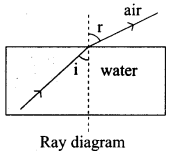When a ray of light travels from dense medium to rarer medium [from water medium to air medium], light ray moves away from the normal.
∴ Angle of incidence < Angle of refraction.

Question 2.
When a ray of light passes from air into glass, is the angle of refraction greater than or less than the angle of incidence?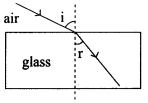When a ray of light travels from rare (air) medium to dense [glass] medium, light ray moves towards the normal.
∴ Angle of refraction < Angle of incidence.

Question 3.
What do you conclude about the speed of light in diamond if the refractive index of diamond is 2.41? way from the mirror. Does the image become smaller or larger? What do you observe?
µ = 2.41; Ca = 3 × 108ms [Velocity of light]
µ = $$\frac{\text { Speed of light in air }}{\text { Speed of light in diamond }}=\frac{\mathrm{C}_{\mathrm{a}}}{\mathrm{C}_{\mathrm{d}}}$$
Cd = $$\frac{C_{a}}{\mu}=\frac{3 \times 10^{8}}{2.41}$$ = 1. 245 × 108 m/s
∴ Speed of light decreases when the light ray travels from air to diamond.

ACTIVITY

Question 1.
Stand before the mirror in your dressing table or the mirror fixed in a steel almirah. Do you see your whole body?
To see your entire body in a mirror, the mirror should be atleast half of your height.
Height of the mirror = Your height/2.

Question 2.
Hold a concave mirror in your hand (or place it in a stand). Direct its reflecting surface towards the sun. Direct the light reflected by the mirror onto a sheet of paper held not very far from the mirror. Move the sheet of paper back and forth gradually until you find a bright, sharp spot of light on the paper. Position the mirror and the paper at the same location for few moments. What do you observe? Why does the paper catches fire?
The concave mirror focus the sunlight at one particular point. At that particular point, amount of sunlight is converged and heat is trapped at that point. This is because, it bum the paper on which the image is focused.

Question 3.
Take a convex mirror. Hold it in one hand. Hold a pencil close to the mirror in the upright position in the other hand. Observe the image of the pencil in the mirror. Is the image erect or inverted? Is it diminished or enlarged? Move the pencil slowly away from the mirror. Does the image become smaller or larger? What do you observe?
When we hold a pencil in the upright position in front of a convex mirror we observe the image of the pencil at the back of the mirror. The image is erect, virtual and smaller in size than the object. As the pencil is moved away from the mirror, the image becomes smaller. When the object is moved away from the mirror, the image would move closer to the focus of the mirror.

Question 4.
Refraction of light at air – water interface
Put a straight pencil into a tank of water or beaker of water at an angle c 45° and look at it from one side and above. How does the pencil look now?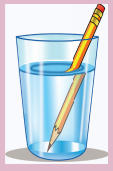The pencil appears to be bent at the surface of water.

### Samacheer Kalvi 9th Science Light In Text Problems

Question 1.
Find the size, nature and position of the image formed when an object of size 1 cm is placed at a distance of 15 cm from a concave mirror of focal length 10 cm.
Solution:
Object distance, u = – 15 cm (to the left of mirror)
Image distance, v = ?
Focal length,f = – 10 cm (concave mirror)
Using mirror formula,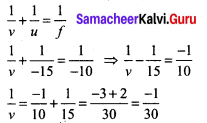Thus, image distance, v = – 30 cm (negative sign indicates that the image is on the left side of the mirror).
∴ Position of image is 30 cm in front of the mirror. Since the image is in front of the mirror, it is real and inverted.
To find the size of the image, we have to calculate the magnification.
m = $$\frac{-v}{u}=\frac{-(-30)}{(-15)}$$ = – 2
We know that, m = $$\frac{h_{2}}{h_{1}}$$
Here, height of the object h1 = 1 cm
– 2 = $$\frac{h_{2}}{1}p.$$
h2 = – 2 × 1 = – 2 cm
The height of image is 2 cm (negative sign shows that the image is formed below the principal axis).

Question 2.
An object 2 cm high is placed at a distance of 16 cm from a concave mirror which produces a real image 3 cm high. Find the position of the image.
Solution:
Height of object h1 = 2 cm
Height of real image h2 = – 3 cm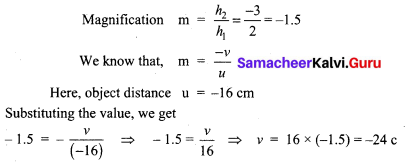The position of image is 24 cm in front of the mirror (negative sign indicates that the image is on the left side of the mirror).

Question 3.
A car is fitted with a convex mirror of focal length 20 cm. Another car is 6 m away from the first car. Find the position of the second car as seen in the mirror of the first. What is the size of the image if the second car is 2 m broad and 1.6 m high?
Solution:
Focal length = 20 cm (convex mirror)
Object distance = – 6m = – 600 cm
Image distance v =?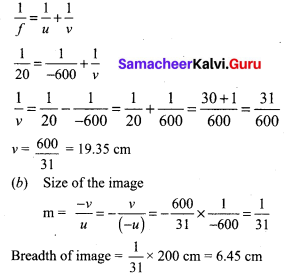Height of image = $$\frac{1}{31}$$ × 160 cm = 5.16 cm

Question 4.
The speed of light in air is 3 × 108 ms– 1 and in glass it is 2 × 108 ms– 1. What is the
refractive index of glass?
Solution:
$$_{a} \mu_{g}=\frac{3 \times 10^{8}}{2 \times 10^{8}}=\frac{3}{2}$$ = 1.5

Question 5.
Light travels from a rarer medium to a denser medium. The angles of incidence and refraction are respectively 45° and 30°. Calculate the refractive index of the second medium with respect to the first medium.
Solution:
Angle of incidence i = 45° ; Angle of refraction r = 30°
$$_{1} \mu_{2}=\frac{\sin i}{\sin r}=\frac{\sin 45^{\circ}}{\sin 30^{\circ}}=\frac{1 / \sqrt{2}}{1 / 2}=\sqrt{2}$$ = 1.414